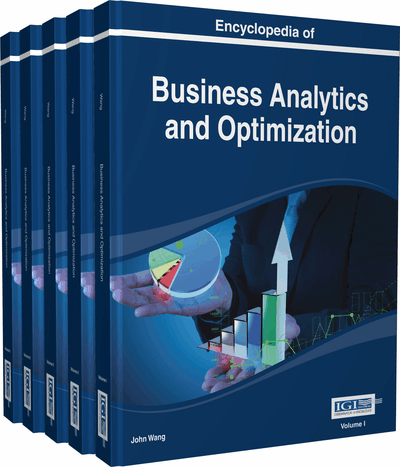# Explaining Predictive Model Decisions

Marko Robnik-Šikonja (Faculty of Computer and Information Science, University of Ljubljana, Slovenia) and Erik Štrumbelj (Faculty of Computer and Information Science, University of Ljubljana, Slovenia)
DOI: 10.4018/978-1-4666-5202-6.ch085

Top

## Background

In a typical problem setting, users are concerned with both prediction accuracy and the interpretability of the prediction model. Complex models have potentially higher accuracy but are more difficult to interpret. This can be alleviated either by sacrificing some prediction accuracy for a more transparent model or by using an explanation method that improves the interpretability of the model. Explaining predictions is straightforward for symbolic models such as decision trees, decision rules, and inductive logic programming, where the models give an overall transparent knowledge in a symbolic form. Therefore, to obtain the explanations of predictions, one simply has to read the rules in the corresponding model. Whether such an explanation is comprehensive in the case of large trees and rule sets is questionable.

For non-symbolic models there are no such straightforward explanations. A lot of effort has been invested into increasing the interpretability of complex models such as ANN (d'Avila Garcez et al., 2001; Palade et al., 2001). For a good review of neural network explanation methods we refer the reader to Jacobsson (2005). For Support Vector Machines interesting approaches are proposed by Hamel (2006) and Poulet (2004). Many approaches exploit the essential property of additive classifiers to provide more comprehensible explanations and visualizations (Jakulin et al., 2005; Mozina et al., 2004; Poulin et al., 2006). Some explanations methods (including the ones presented here) are more general in a sense that they can be used with any type of classification model (Lemaire et al., 2008; Robnik-Šikonja & Kononenko, 2008; Štrumbelj et al., 2010). This enables their application with almost any prediction model and allows users to analyse and compare outputs of different analytical techniques.

## Key Terms in this Chapter

Attribute Evaluation: A data mining procedure which estimates the utility of attributes for given task (usually prediction). Attribute evaluation is used in many data mining tasks, for example in feature subset selection, feature weighting, feature ranking, feature construction, decision and regression tree building, data discretization, visualization, and comprehension.

Marginal Effect of a Feature: Is a difference between model’s prediction with the feature and without it, holding all other features intact.

Model Level Explanation: Aims is to make transparent the prediction process of a particular model. Empirical observations show that better models (with higher prediction accuracy) enable better explanation at the domain level.

Feature Contribution: A value assigned to a feature (or its value) that is proportional to the feature’s share in the model’s prediction for an instance.

Instance Level Explanation: Explanation of prediction of a single instance with a given model, that is, at the model level.

Domain Level Explanation: Tries to find the true causal relationship between the dependent and independent variables. Typically this level is unreachable unless we are dealing with artificial domains where all the relations as well as the probability distributions are known in advance.

Quality of the Explanation: Can be judged by several criteria: accuracy (generalization ability), fidelity (how well the explanation reflects behavior of the model), consistency (similarity of behavior for different models trained on the same task), and comprehensibility (readability and size of the extracted knowledge).

Expressive Power of Explanation: Describes the language of extracted knowledge: propositional logic (that is, if-then rules), nonconventional logic (for example, fuzzy logic), first-order logic, and finite state machines (deterministic, nondeterministic, stochastic).

Portability of Explanation: Describes how well the explanation technique covers the set of available models.

## Complete Chapter List

Search this Book:
Reset